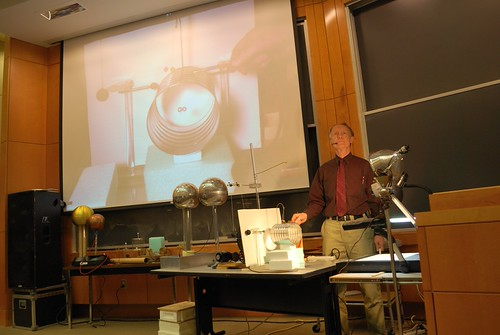Home » Posts tagged 'ELECTROSTATICS'

# Tag Archives: ELECTROSTATICS

### Visitors

• 4,226,099 hits

### Subscribe to PlusTwoPhysics via Email

Join 4,744 other subscribers

## Question from Electrostatics

A charge ‘q’is placed at the centre of the line joining two equal charges Q.The system of 3 charges will be in equilibrium, if ‘q’is equal to;

a)-Q/2

b)-Q/4

c) Q/2

d) Q/4

The answer is (b)  – Q / 4

For the three charges to be in equilibrium, the net force must be zero. i.e, considering the net force on any one of Q (since q will be in equilibrium for any value of q when placed at the midpoint)## JEE Advanced – Study Materials

02-Electrostatics

03-Current-Electricity

04-Electromagnetism

05-Mechanics

06-Optics

07-HeatAndThermodynamics

08-Sound

09-ModernPhysics

10-PropertiesOfMatter## Electrostatics – One mark and two marks questions for practice

So far we were concentrating on 3 and five marks questions only. Now I think that concentrating on one and two marks questions will further increase the chance of scoring better.

It’s true that just by concentrating on the three marks and five marks questions one can easily score greater than 60% marks. But it is not enough. When you CAN SCORE MORE why should you compromise for less?

Often the one and two marks questions are tricky and twisted. But, when the questions are tricky, there is a greater chance to score. All you need is to apply your commonsense (often complained that it is not so common!) and logical reasoning. The basic facts remain the same however the question maker twist them. So, believe that whatever question asked are based on what you have learnt. But beware!, whatever conclusions you draw must satisfy your commonsense as well as that of the examiner.

So, here are a collection of one mark and two marks questions from ELECTROSTATICS. The straight – forward questions are intentionally avoided.

Electrostatics 1

Electrostatics 2

Electric Potential

Related articles## Class 12 Physics : Electrostatics Revision Test

Max marks:50 Time:2hrs

1. A glass rod rubbed with silk acquires a charge of 6×10-12 C. Calculate the number of electrons it has gained or lost. (1)
2. If the magnitude of each charge becomes doubled, the separation between them is halved and the system of charges kept in a medium of=3 Calculate the force between them. (1)
3. If two equal charges separated by a distance of 2m in air medium experiences a force of 0.9×1010 C, Calculate the magnitude of each charge. (1)
4. Electric field intensity is 400Vm-1 at a distance of 2 m from a point charge. At what distance it will become 100 Vm-1? (1)
5. What will be the force experienced by a particle of charge 2µC placed in an electric field of 10kVm-1? (1)
6. What is the work done by moving 400μF charge between two points separated by a distance of 27 cm on an equipotential surface? (1)
7. Calculate the number of electric lines of force from a charge 1.7708X10-6 C in air medium? (1)
8. Two protons ‘A’ and ‘B’ are placed between two parallel plates having a potential difference ‘V’ as shown in the figure. Will these protons experience equal or unequal force? (1)
9. Draw an equipotential surface for a point charge q> 0(1)
10. The capacitance of a parallel plate capacitor is ‘C’. If 15 such capacitors are connected in series and then in parallel, what is the ratio of effective capacitance of first case to the second? (1)
11. What is electric polarization ,polar and non polar molecules?( 2)
12. 2) Define electrostatic induction and corona discharge. ( 2)
13. Calculate the ratio of force experienced by a proton to that of the electron placed in an electric field of 4KVm-1. ( 2)
14. If an electrician has three capacitors of capacitances 4µF, 7µF& 12µF. How should he connect them, So that the effective capacitance will be 10µF? ( 2)
15. Define the SI unit of potential difference and charge. ( 2)
16. Two charges of 20µC and -20µC are placed 20 cm apart at points A and B respectively. Compute the electric field strength at point C, 20 cm away from both A and B.(3)
17. Three capacitors of capacitances 4µF, 6µF and 8µF are connected parallel to each other and the combination is connected across a battery of 25V. Calculate the charges present on each capacitor and the total charge flows from the source. (3)
18. Derive an expression for the torque experienced by a dipole in an external electric field. (3)
19. Obtain an expression for the capacitance of a parallel plate capacitor. (3)
20. Derive the relation E=(3)
21. Derive an expression for the potential at a point due to dipole. Write the special cases also.(5)
22. Derive an expression for ‘E’ at a point on the axial line of the dipole. (5)
23. Explain with a neat diagram the principle, construction and working of a Van de Graff generator. (5)

*************************************

## Electrostatics Question Banks

Objective Type Questions from Electrostatics

Electrostatics Question Bank from TutorNext

Electrostatics Question Bank from 2lv.in

## Electric Flux

From: Nancy James

Subject: Electric flux
Message Body:
Could some one please explain what is written in the ncert book of PHYSICs class XII about ‘electric flux’?Save the images, open and zoomin to read